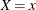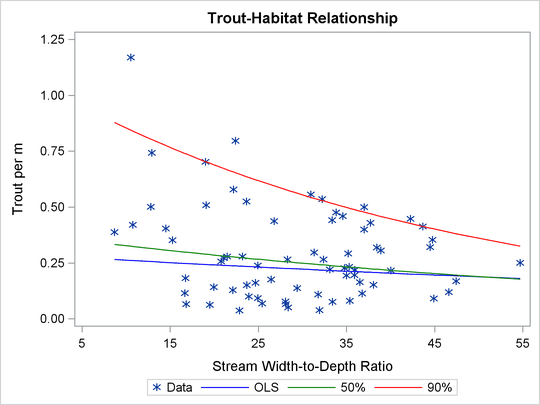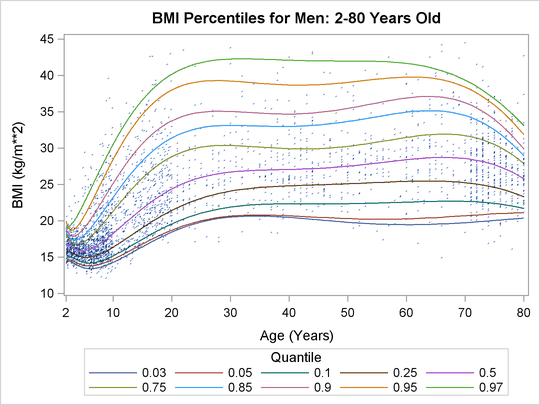## Overview: QUANTREG Procedure

The QUANTREG procedure models the effects of covariates on the conditional quantiles of a response variable by means of quantile regression.

Ordinary least squares (OLS) regression models the relationship between one or more covariates X and the conditional mean of the response variable Y given. Quantile regression, which was introduced by Koenker and Bassett (1978), extends the regression model to conditional quantiles of the response variable, such as the median or the 90th percentile. Quantile regression is particularly useful when the rate of change in the conditional quantile, expressed by the regression coefficients, depends on the quantile.

Figure 77.1: Trout Density in StreamsFigure 77.1 illustrates an ecological study in which it is revealing to model upper conditional quantiles. The points represent measurements of trout density and stream width-to-depth ratio taken at 13 streams over seven years.

As analyzed by Dunham, Cade, and Terrell (2002), in addition to the ratio, trout density depends on a number of unmeasured limiting factors related to the integrity of stream habitat. The interaction of these factors results in unequal variances for the conditional distributions of density given the ratio. When the ratio is the active limiting effect, changes in the upper conditional percentiles of density provide a better estimate of this effect than changes in the conditional mean.

The red and green curves represent the conditional 90th and 50th percentiles of density as determined with the QUANTREG procedure. The analysis was done by using a simple linear regression model for the logarithm of density. (The curves in Figure 77.1 were obtained by transforming the fitted lines back to the original scale. For more details, see the section Analysis of Fish-Habitat Relationships.) The slope parameter for the 90th percentile has an estimated value of –0.0215 and is significant with a p-value less than 0.01. On the other hand, the slope parameter for the 50th percentile is not significantly different from zero. Similarly, the slope parameter for the mean, obtained with OLS regression, is not significantly different from zero.

Figure 77.2: Quantiles for Body Mass IndexQuantile regression is especially useful with data that are heterogeneous in the sense that the tails and the central location of the conditional distributions vary differently with the covariates. An even more pronounced example of heterogeneity is shown in Figure 77.2, which plots the body mass index of 8,250 men versus their age.

Here, both upper (overweight) and lower (underweight) conditional quantiles are important because they provide the basis for developing growth charts and establishing health standards. The curves in Figure 77.2 were determined with the QUANTREG procedure by using polynomial quantile regression; details are provided in the section Growth Charts for Body Mass Index. Clearly, the rate of change with age (as expressed by the regression coefficients), particularly for ages less than 20, is different for each conditional quantile.

Heterogeneous data occur in many fields, including biomedicine, econometrics, survival analysis, and ecology. Quantile regression, which includes median regression as a special case, provides a complete picture of the covariate effect when a set of percentiles is modeled, and so it offers the capability to capture important features of the data that might be missed by models that average over the conditional distribution.

Because it makes no distributional assumption about the error term in the model, quantile regression offers considerable model robustness. The assumption of normality, which is often made with OLS regression in order to compute conditional quantiles as offsets from the mean, forces a common set of regression coefficients for all the quantiles. Obviously, quantiles with common slopes would be inappropriate in the preceding examples.

Quantile regression is also flexible in the sense that it does not involve a link function that relates the variance and the mean of the response variable. Generalized linear models, which you can fit with the GENMOD procedure, require both a link function and a distributional assumption such as the normal or Poisson distribution. The goal of generalized linear models is inference about the regression parameters in the linear predictor for the mean of the population. In contrast, the goal of quantile regression is inference on regression coefficients for the conditional quantiles of a response variable that is usually assumed to be continuous.

Quantile regression also offers a degree of data robustness. Unlike OLS regression, it is robust to extreme points in the response direction (outliers). However, it is not robust to extreme points in the covariate space (leverage points). When both types of robustness are of concern, you should consider using the ROBUSTREG procedure (Chapter 80: The ROBUSTREG Procedure.)

Also, unlike OLS regression, quantile regression is equivariant to monotone transformations of the response variable. For instance, as illustrated in the trout example, the logarithm of the 90th conditional percentile of trout density is the 90th conditional percentile of the logarithm of density.

Note that quantile regression cannot be carried out simply by segmenting the unconditional distribution of the response variable and then obtaining least squares fits for the subsets. This approach leads to disastrous results when, for example, the data include outliers. In contrast, quantile regression uses all of the data for fitting quantiles, even the extreme quantiles.